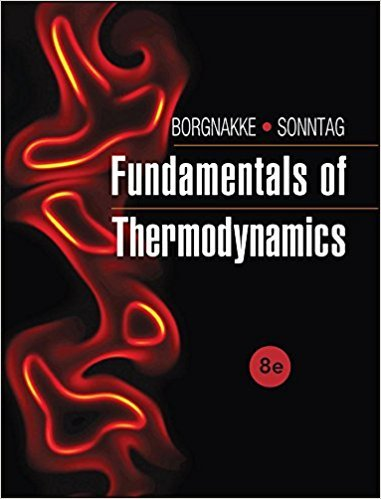×
Get Full Access to Fundamentals Of Thermodynamcs - 8 Edition - Chapter 3 - Problem 71hp
Get Full Access to Fundamentals Of Thermodynamcs - 8 Edition - Chapter 3 - Problem 71hp

×

# Find the phase and the missing properties of P, T, v, u,ISBN: 9781118131992 58

## Solution for problem 71HP Chapter 3

Fundamentals of Thermodynamcs | 8th Edition

• Textbook Solutions
• 2901 Step-by-step solutions solved by professors and subject experts
• Get 24/7 help from StudySoup virtual teaching assistantsFundamentals of Thermodynamcs | 8th Edition

4 5 1 271 Reviews
16
0
Problem 71HP

Problem 71HP

Find the phase and the missing properties of P, T, v, u, and x for water at

a. 500 kPa, 100°C

b. 5000 kPa, u = 800 kJ/kg

c. 5000 kPa, v = 0.06 m3/kg

d. −6°C, v = 1 m3/kg

Step-by-Step Solution:

Solution 71HP

Part (a)

Step 1 of 8:

We are going to find the specific volume, specific internal energy and the quality of water at the given pressure and temperature.

The given pressure P = 500 kPa = 0.5 MPa

The given temperature T = 100°C

Step 2 of 6

Step 3 of 6

##### ISBN: 9781118131992

Unlock Textbook Solution